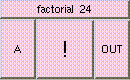FACTORIALTHE BOX'S MAIN HELP: Returns the factorial of A. OUT = 1 * 2 * 3 .. A A (input): The factorial input value. This value should be a positive integer. Values <= 1 yield 1. OUT (output): Output is the factorial of the input. OUT = 1 * 2 * 3 ..on thru the value of 'A'.

<-- BACK TO Math